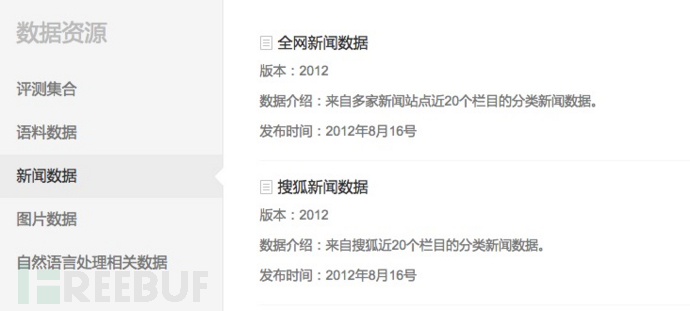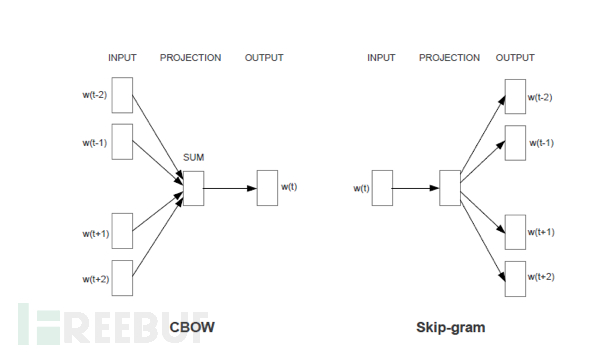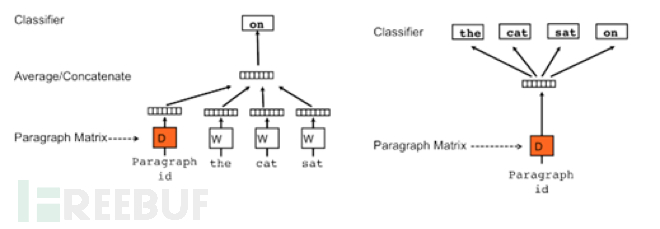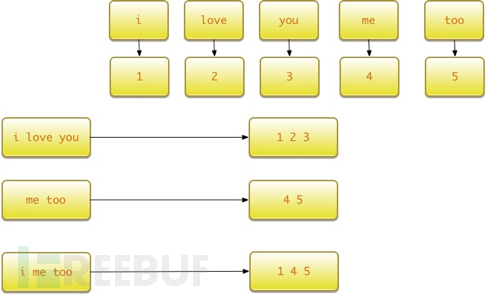# 训练语料

word2vec的算法是公开的，word2vec模型的质量完全取决于训练语料的质量。目前免费开放的预料不多，中文语料更是凤毛麟角。```http://www.sogou.com/labs/resource/cs.php
```

## 数据格式

```<doc>

<url>页面URL</url>

<docno>页面ID</docno>

<contenttitle>页面标题</contenttitle>

<content>页面内容</content>

</doc>
```

## 数据文件

• 迷你版(样例数据, 110KB)：tar.gz格式，zip格式

• 完整版(648MB)：tar.gz格式，zip格式

• 历史版本：2008版(6KB)：完整版(同时提供硬盘拷贝,65GB)：tar.gz格式

# 数据预处理

## 提取中文内容

```tar -zxvf news_sohusite_xml.full.tar.gz
cat news_sohusite_xml.dat | iconv -f gb18030 -t utf-8 | grep "<content>" > news_sohusite.txt
sed -i "" 's/<content>//g' news_sohusite.txt
sed -i "" 's/<\/content>//g' news_sohusite.txt
```

```iconv -f encoding [-t encoding] [inputfile]...
```

• -f encoding :把字符从encoding编码开始转换。
• -t encoding :把字符转换到encoding编码。
• -l :列出已知的编码字符集合
• -o file :指定输出文件
• -c :忽略输出的非法字符
• -s :禁止警告信息，但不是错误信息
• –verbose :显示进度信息
• -f和-t所能指定的合法字符在-l选项的命令里面都列出来了

## 中文切词

```python -m jieba -d ' ' news_sohusite.txt > news_sohusite_cutword.txt
```

# 训练word2vec

```def train_word2vec(filename):
#模型文件不存在才处理
if not os.path.exists(word2vec_file):
sentences = LineSentence(filename)
#sg=0 使用cbow训练, sg=1对低频词较为敏感
model = Word2Vec(sentences,
size=n_dim, window=5, min_count=2, sg=1, workers=2)
model.save(word2vec_file)
```

Word2Vec函数常见的几个参数含义如下：

• sentences表示需要处理的语料
• size表示word2vec的维数，一般50-300
• window表示处理word时的窗口长度
• min_count表示处理分析的word出现的最小次数
• sg为1表示使用skip-gram算法，为0为cbow
• workers表示计算使用的线程数
• iter表示迭代计算的次数

# 使用word2vec处理中文

```def buildWordVector(imdb_w2v,text, size):
vec = np.zeros(size).reshape((1, size))
count = 0.
#print text
for word in text.split():
#print word
try:
vec += imdb_w2v[word].reshape((1, size))
count += 1.
except KeyError:
print word
continue
if count != 0:
vec /= count
return vec
```

```#加载训练好的词向量模型

x_vecs = np.concatenate([buildWordVector(model,z, n_dim) for z in x])
x_vecs = scale(x_vecs)
```

# 测试效果

## 寻找近义词

### 百度的近义词

```print pd.Series(model.most_similar(u'百度'))
0      (网易, 0.844283640385)
1    (搜索引擎, 0.822018146515)
2      (腾讯, 0.774820387363)
3       (搜狗, 0.76777946949)
4      (新浪, 0.760137319565)
5      (奇虎, 0.745484173298)
6      (文库, 0.725166857243)
7    (手机软件, 0.717750906944)
8       (优酷, 0.70574760437)
9      (客户端, 0.70448333025)
```

### 微信的近义词

```print pd.Series(model.most_similar(u'微信'))
0     (摇一摇, 0.768034994602)
1      (陌陌, 0.763847649097)
2    (网上聊天, 0.751431167126)
3    (聊天工具, 0.731707036495)
4      (盗号, 0.722806692123)
5      (飞聊, 0.715048789978)
6      (手机, 0.706719994545)
7     (发短信, 0.704942345619)
8      (聊天, 0.691777765751)
9    (账号密码, 0.679741084576)
```

## 单词运算

word2vec的一个神奇之处就是把文字转换成了数字，数字之间的加减运算，同样适用于word2vec。

### 足球+明星

```print pd.Series(model.most_similar(positive=[u'足球'+u'明星']))
0      (巨星, 0.741350233555)
1    (光芒万丈, 0.727712750435)
2     (和亨利, 0.722848057747)
3      (球星, 0.722578346729)
4       (已贵, 0.71345859766)
5     (格米利, 0.694822609425)
6     (支斯篮, 0.690492749214)
7      (田坛, 0.689639627934)
8      (体坛, 0.689606904984)
9     (竞神锋, 0.684816122055)
```

### 球星-明星

```print pd.Series(model.most_similar(positive=[u'球星'],negative=[u'明星']))
dtype: object
0    (国际米兰, 0.492849290371)
1      (中锋, 0.480526059866)
2      (球员, 0.479797780514)
3     (上赛季, 0.479528963566)
4      (主帅, 0.479275196791)
5      (球队, 0.477513790131)
6     (德里奇, 0.474446773529)
7     (热那亚, 0.472252100706)
8      (中场, 0.459134191275)
9       (巴萨, 0.45858669281)
```

## 比较单词的相似度

### 比较微信和陌陌

```print model.wv.similarity(u'微信', u'陌陌')
0.763847656891
```

### 比较男人和坏人

```print model.wv.similarity(u'男人', u'坏人')
0.617036796702
```

# 训练语料

word2vec的算法是公开的，word2vec模型的质量完全取决于训练语料的质量。目前免费开放的预料不多，中文语料更是凤毛麟角。```http://www.sogou.com/labs/resource/cs.php
```

## 数据格式

```<doc>

<url>页面URL</url>

<docno>页面ID</docno>

<contenttitle>页面标题</contenttitle>

<content>页面内容</content>

</doc>
```

## 数据文件

• 迷你版(样例数据, 110KB)：tar.gz格式，zip格式

• 完整版(648MB)：tar.gz格式，zip格式

• 历史版本：2008版(6KB)：完整版(同时提供硬盘拷贝,65GB)：tar.gz格式

# 数据预处理

## 提取中文内容

```tar -zxvf news_sohusite_xml.full.tar.gz
cat news_sohusite_xml.dat | iconv -f gb18030 -t utf-8 | grep "<content>" > news_sohusite.txt
sed -i "" 's/<content>//g' news_sohusite.txt
sed -i "" 's/<\/content>//g' news_sohusite.txt
```

```iconv -f encoding [-t encoding] [inputfile]...
```

• -f encoding :把字符从encoding编码开始转换。
• -t encoding :把字符转换到encoding编码。
• -l :列出已知的编码字符集合
• -o file :指定输出文件
• -c :忽略输出的非法字符
• -s :禁止警告信息，但不是错误信息
• –verbose :显示进度信息
• -f和-t所能指定的合法字符在-l选项的命令里面都列出来了

## 中文切词

```python -m jieba -d ' ' news_sohusite.txt > news_sohusite_cutword.txt
```

# 训练word2vec

```def train_word2vec(filename):
#模型文件不存在才处理
if not os.path.exists(word2vec_file):
sentences = LineSentence(filename)
#sg=0 使用cbow训练, sg=1对低频词较为敏感
model = Word2Vec(sentences,
size=n_dim, window=5, min_count=2, sg=1, workers=2)
model.save(word2vec_file)
```

Word2Vec函数常见的几个参数含义如下：

• sentences表示需要处理的语料
• size表示word2vec的维数，一般50-300
• window表示处理word时的窗口长度
• min_count表示处理分析的word出现的最小次数
• sg为1表示使用skip-gram算法，为0为cbow
• workers表示计算使用的线程数
• iter表示迭代计算的次数

# 使用word2vec处理中文

```def buildWordVector(imdb_w2v,text, size):
vec = np.zeros(size).reshape((1, size))
count = 0.
#print text
for word in text.split():
#print word
try:
vec += imdb_w2v[word].reshape((1, size))
count += 1.
except KeyError:
print word
continue
if count != 0:
vec /= count
return vec
```

```#加载训练好的词向量模型

x_vecs = np.concatenate([buildWordVector(model,z, n_dim) for z in x])
x_vecs = scale(x_vecs)
```

# 测试效果

## 寻找近义词

### 百度的近义词

```print pd.Series(model.most_similar(u'百度'))
0      (网易, 0.844283640385)
1    (搜索引擎, 0.822018146515)
2      (腾讯, 0.774820387363)
3       (搜狗, 0.76777946949)
4      (新浪, 0.760137319565)
5      (奇虎, 0.745484173298)
6      (文库, 0.725166857243)
7    (手机软件, 0.717750906944)
8       (优酷, 0.70574760437)
9      (客户端, 0.70448333025)
```

### 微信的近义词

```print pd.Series(model.most_similar(u'微信'))
0     (摇一摇, 0.768034994602)
1      (陌陌, 0.763847649097)
2    (网上聊天, 0.751431167126)
3    (聊天工具, 0.731707036495)
4      (盗号, 0.722806692123)
5      (飞聊, 0.715048789978)
6      (手机, 0.706719994545)
7     (发短信, 0.704942345619)
8      (聊天, 0.691777765751)
9    (账号密码, 0.679741084576)
```

## 单词运算

word2vec的一个神奇之处就是把文字转换成了数字，数字之间的加减运算，同样适用于word2vec。

### 足球+明星

```print pd.Series(model.most_similar(positive=[u'足球'+u'明星']))
0      (巨星, 0.741350233555)
1    (光芒万丈, 0.727712750435)
2     (和亨利, 0.722848057747)
3      (球星, 0.722578346729)
4       (已贵, 0.71345859766)
5     (格米利, 0.694822609425)
6     (支斯篮, 0.690492749214)
7      (田坛, 0.689639627934)
8      (体坛, 0.689606904984)
9     (竞神锋, 0.684816122055)
```

### 球星-明星

```print pd.Series(model.most_similar(positive=[u'球星'],negative=[u'明星']))
dtype: object
0    (国际米兰, 0.492849290371)
1      (中锋, 0.480526059866)
2      (球员, 0.479797780514)
3     (上赛季, 0.479528963566)
4      (主帅, 0.479275196791)
5      (球队, 0.477513790131)
6     (德里奇, 0.474446773529)
7     (热那亚, 0.472252100706)
8      (中场, 0.459134191275)
9       (巴萨, 0.45858669281)
```

## 比较单词的相似度

### 比较微信和陌陌

```print model.wv.similarity(u'微信', u'陌陌')
0.763847656891
```

### 比较男人和坏人

```print model.wv.similarity(u'男人', u'坏人')
0.617036796702
```

# Word2VecCBOW和Skip-gram原理图

CBOW模型能够根据输入周围n-1个词来预测出这个词本身，而Skip-gram模型能够根据词本身来预测周围有哪些词。也就是说，CBOW模型的输入是某个词A周围的n个单词的词向量之和，输出是词A本身的词向量，而Skip-gram模型的输入是词A本身，输出是词A周围的n个单词的词向量。Word2Vec最常用的开源实现之一就是gensim，网址为:

gensim的安装非常简单：

gensim的使用非常简洁，加载数据和训练数据可以合并，训练好模型后就可以按照单词获取对应的向量表示：

sentences = [['first', 'sentence'], ['second', 'sentence']]model = gensim.models.Word2Vec(sentences, min_count=1)print model['first']

```model = Word2Vec(sentences, min_count=10)
```

size=200

```model = Word2Vec(sentences, size=200)
```

```model=gensim.models.Word2Vec(size=200, window=8, min_count=10, iter=10, workers=cores)
```

```cores=multiprocessing.cpu_count()
```

```x=x_train+x_testmodel.build_vocab(x)
model.train(x, total_examples=model.corpus_count, epochs=model.iter)
```

```model[“love”]
```

Word2Vec的维度与之前设置的神经网络的隐藏层的单元数相同为200，也就是说是一个长度为200的一维向量。通过遍历一段英文，逐次获取每个单词对应的Word2Vec，连接起来就可以获得该英文段落对应的Word2Vec：

```def getVecsByWord2Vec(model, corpus, size):
x=[]
for text in corpus:
xx = []
for i, vv in enumerate(text):
try:
xx.append(model[vv].reshape((1,size)))
except KeyError:
continue
x = np.concatenate(xx)
x=np.array(x, dtype='float')
return x
```

# Doc2VecDM和DBOW原理图

```model=Doc2Vec(dm=0, dbow_words=1, size=max_features, window=8, min_count=10, iter=10, workers=cores)
```

```SentimentDocument = namedtuple('SentimentDocument', 'words tags')
```

```def labelizeReviews(reviews, label_type):
labelized = []
for i, v in enumerate(reviews):
label = '%s_%s' % (label_type, i)
labelized.append(SentimentDocument(v, [label]))
return labelized
```

```x=x_train+x_test
model.build_vocab(x)
model.train(x, total_examples=model.corpus_count, epochs=model.iter)
```

```model.docvecs[”I love tensorflow”]
```

```array([ 0.02664499, 0.00475204, -0.03981256, 0.03796276, -0.03206162, 0.10963056, -0.04897128, 0.00151982, -0.03258783, 0.04711508, -0.00667155, -0.08523653, -0.02975186, 0.00166316, 0.01915652, -0.03415785, -0.05794788, 0.05110953, 0.01623618, -0.00512495, -0.06385455, -0.0151557 , 0.00365376, 0.03015811, 0.0229462 , 0.03176891, 0.01117626, -0.00743352, 0.02030453, -0.05072152, -0.00498496, 0.00151227, 0.06122205, -0.01811385, -0.01715777, 0.04883198, 0.03925886, -0.03568915, 0.00805744, 0.01654406, -0.05160677, 0.0119908 , -0.01527433, 0.02209963, -0.10316766, -0.01069367, -0.02432527, 0.00761799, 0.02763799, -0.04288232], dtype=float32)
```

Doc2Vec的维度与之前设置的神经网络的隐藏层的单元数相同为200，也就是说是一个长度为200的一维向量。以英文段落为单位，通过遍历训练数据集和测试数据集，逐次获取每个英文段落对应的Doc2Vec，这里的英文段落就可以理解为数据集中针对电影的一段评价：

```def getVecs(model, corpus, size):
vecs = [np.array(model.docvecs[z.tags]).reshape((1, size)) for z in corpus]
return np.array(np.concatenate(vecs),dtype='float')
```

```if os.path.exists(doc2ver_bin):
print "Find cache file %s" % doc2ver_bin
else:
model=Doc2Vec(size=max_features, window=5, min_count=2, workers=cores,iter=40)
model.build_vocab(x))
model.train(x, total_examples=model.corpus_count, epochs=model.iter)
model.save(doc2ver_bin)
```# Word2VecCBOW和Skip-gram原理图

CBOW模型能够根据输入周围n-1个词来预测出这个词本身，而Skip-gram模型能够根据词本身来预测周围有哪些词。也就是说，CBOW模型的输入是某个词A周围的n个单词的词向量之和，输出是词A本身的词向量，而Skip-gram模型的输入是词A本身，输出是词A周围的n个单词的词向量。Word2Vec最常用的开源实现之一就是gensim，网址为:

gensim的安装非常简单：

gensim的使用非常简洁，加载数据和训练数据可以合并，训练好模型后就可以按照单词获取对应的向量表示：

sentences = [['first', 'sentence'], ['second', 'sentence']]model = gensim.models.Word2Vec(sentences, min_count=1)print model['first']

```model = Word2Vec(sentences, min_count=10)
```

size=200

```model = Word2Vec(sentences, size=200)
```

```model=gensim.models.Word2Vec(size=200, window=8, min_count=10, iter=10, workers=cores)
```

```cores=multiprocessing.cpu_count()
```

```x=x_train+x_testmodel.build_vocab(x)
model.train(x, total_examples=model.corpus_count, epochs=model.iter)
```

```model[“love”]
```

Word2Vec的维度与之前设置的神经网络的隐藏层的单元数相同为200，也就是说是一个长度为200的一维向量。通过遍历一段英文，逐次获取每个单词对应的Word2Vec，连接起来就可以获得该英文段落对应的Word2Vec：

```def getVecsByWord2Vec(model, corpus, size):
x=[]
for text in corpus:
xx = []
for i, vv in enumerate(text):
try:
xx.append(model[vv].reshape((1,size)))
except KeyError:
continue
x = np.concatenate(xx)
x=np.array(x, dtype='float')
return x
```

# Doc2VecDM和DBOW原理图

```model=Doc2Vec(dm=0, dbow_words=1, size=max_features, window=8, min_count=10, iter=10, workers=cores)
```

```SentimentDocument = namedtuple('SentimentDocument', 'words tags')
```

```def labelizeReviews(reviews, label_type):
labelized = []
for i, v in enumerate(reviews):
label = '%s_%s' % (label_type, i)
labelized.append(SentimentDocument(v, [label]))
return labelized
```

```x=x_train+x_test
model.build_vocab(x)
model.train(x, total_examples=model.corpus_count, epochs=model.iter)
```

```model.docvecs[”I love tensorflow”]
```

```array([ 0.02664499, 0.00475204, -0.03981256, 0.03796276, -0.03206162, 0.10963056, -0.04897128, 0.00151982, -0.03258783, 0.04711508, -0.00667155, -0.08523653, -0.02975186, 0.00166316, 0.01915652, -0.03415785, -0.05794788, 0.05110953, 0.01623618, -0.00512495, -0.06385455, -0.0151557 , 0.00365376, 0.03015811, 0.0229462 , 0.03176891, 0.01117626, -0.00743352, 0.02030453, -0.05072152, -0.00498496, 0.00151227, 0.06122205, -0.01811385, -0.01715777, 0.04883198, 0.03925886, -0.03568915, 0.00805744, 0.01654406, -0.05160677, 0.0119908 , -0.01527433, 0.02209963, -0.10316766, -0.01069367, -0.02432527, 0.00761799, 0.02763799, -0.04288232], dtype=float32)
```

Doc2Vec的维度与之前设置的神经网络的隐藏层的单元数相同为200，也就是说是一个长度为200的一维向量。以英文段落为单位，通过遍历训练数据集和测试数据集，逐次获取每个英文段落对应的Doc2Vec，这里的英文段落就可以理解为数据集中针对电影的一段评价：

```def getVecs(model, corpus, size):
vecs = [np.array(model.docvecs[z.tags]).reshape((1, size)) for z in corpus]
return np.array(np.concatenate(vecs),dtype='float')
```

```if os.path.exists(doc2ver_bin):
print "Find cache file %s" % doc2ver_bin
else:
model=Doc2Vec(size=max_features, window=5, min_count=2, workers=cores,iter=40)
model.build_vocab(x))
model.train(x, total_examples=model.corpus_count, epochs=model.iter)
model.save(doc2ver_bin)```# Word2VecCBOW和Skip-gram原理图

CBOW模型能够根据输入周围n-1个词来预测出这个词本身，而Skip-gram模型能够根据词本身来预测周围有哪些词。也就是说，CBOW模型的输入是某个词A周围的n个单词的词向量之和，输出是词A本身的词向量，而Skip-gram模型的输入是词A本身，输出是词A周围的n个单词的词向量。Word2Vec最常用的开源实现之一就是gensim，网址为:

gensim的安装非常简单：

gensim的使用非常简洁，加载数据和训练数据可以合并，训练好模型后就可以按照单词获取对应的向量表示：

sentences = [['first', 'sentence'], ['second', 'sentence']]model = gensim.models.Word2Vec(sentences, min_count=1)print model['first']

```model = Word2Vec(sentences, min_count=10)
```

size=200

```model = Word2Vec(sentences, size=200)
```

```model=gensim.models.Word2Vec(size=200, window=8, min_count=10, iter=10, workers=cores)
```

```cores=multiprocessing.cpu_count()
```

```x=x_train+x_testmodel.build_vocab(x)
model.train(x, total_examples=model.corpus_count, epochs=model.iter)
```

```model[“love”]
```

Word2Vec的维度与之前设置的神经网络的隐藏层的单元数相同为200，也就是说是一个长度为200的一维向量。通过遍历一段英文，逐次获取每个单词对应的Word2Vec，连接起来就可以获得该英文段落对应的Word2Vec：

```def getVecsByWord2Vec(model, corpus, size):
x=[]
for text in corpus:
xx = []
for i, vv in enumerate(text):
try:
xx.append(model[vv].reshape((1,size)))
except KeyError:
continue
x = np.concatenate(xx)
x=np.array(x, dtype='float')
return x
```

# Doc2VecDM和DBOW原理图

```model=Doc2Vec(dm=0, dbow_words=1, size=max_features, window=8, min_count=10, iter=10, workers=cores)
```

```SentimentDocument = namedtuple('SentimentDocument', 'words tags')
```

```def labelizeReviews(reviews, label_type):
labelized = []
for i, v in enumerate(reviews):
label = '%s_%s' % (label_type, i)
labelized.append(SentimentDocument(v, [label]))
return labelized
```

```x=x_train+x_test
model.build_vocab(x)
model.train(x, total_examples=model.corpus_count, epochs=model.iter)
```

```model.docvecs[”I love tensorflow”]
```

```array([ 0.02664499, 0.00475204, -0.03981256, 0.03796276, -0.03206162, 0.10963056, -0.04897128, 0.00151982, -0.03258783, 0.04711508, -0.00667155, -0.08523653, -0.02975186, 0.00166316, 0.01915652, -0.03415785, -0.05794788, 0.05110953, 0.01623618, -0.00512495, -0.06385455, -0.0151557 , 0.00365376, 0.03015811, 0.0229462 , 0.03176891, 0.01117626, -0.00743352, 0.02030453, -0.05072152, -0.00498496, 0.00151227, 0.06122205, -0.01811385, -0.01715777, 0.04883198, 0.03925886, -0.03568915, 0.00805744, 0.01654406, -0.05160677, 0.0119908 , -0.01527433, 0.02209963, -0.10316766, -0.01069367, -0.02432527, 0.00761799, 0.02763799, -0.04288232], dtype=float32)
```

Doc2Vec的维度与之前设置的神经网络的隐藏层的单元数相同为200，也就是说是一个长度为200的一维向量。以英文段落为单位，通过遍历训练数据集和测试数据集，逐次获取每个英文段落对应的Doc2Vec，这里的英文段落就可以理解为数据集中针对电影的一段评价：

```def getVecs(model, corpus, size):
vecs = [np.array(model.docvecs[z.tags]).reshape((1, size)) for z in corpus]
return np.array(np.concatenate(vecs),dtype='float')
```

```if os.path.exists(doc2ver_bin):
print "Find cache file %s" % doc2ver_bin
else:
model=Doc2Vec(size=max_features, window=5, min_count=2, workers=cores,iter=40)
model.build_vocab(x))
model.train(x, total_examples=model.corpus_count, epochs=model.iter)
model.save(doc2ver_bin)```## 词袋模型

• 词集模型：单词构成的集合，集合自然每个元素都只有一个，也即词集中的每个单词都只有一个。

• 词袋模型：在词集的基础上如果一个单词在文档中出现不止一次，统计其出现的次数（频数）。

``````from sklearn.feature_extraction.text import CountVectorizer
``````

``````vectorizer = CountVectorizer(min_df=1)

vectorizer

CountVectorizer(analyzer=...'word', binary=False, decode_error=...'strict',

dtype=<... 'numpy.int64'>, encoding=...'utf-8', input=...'content',

lowercase=True, max_df=1.0, max_features=None, min_df=1,

ngram_range=(1, 1), preprocessor=None, stop_words=None,

strip_accents=None, token_pattern=...'(?u)\\b\\w\\w+\\b',

tokenizer=None, vocabulary=None)
``````

``````corpus = [

...     'This is the first document.',

...     'This is the second second document.',

...     'And the third one.',

...     'Is this the first document?',

... ]

X = vectorizer.fit_transform(corpus)

X

<4x9 sparse matrix of type '<... 'numpy.int64'>'

with 19 stored elements in Compressed Sparse ... format>
``````

``````>>> vectorizer.get_feature_names() == (

...     ['and', 'document', 'first', 'is', 'one',

...      'second', 'the', 'third', 'this'])

True
``````

``````>>> X.toarray()

array([[0, 1, 1, 1, 0, 0, 1, 0, 1],

[0, 1, 0, 1, 0, 2, 1, 0, 1],

[1, 0, 0, 0, 1, 0, 1, 1, 0],

[0, 1, 1, 1, 0, 0, 1, 0, 1]]...)
``````

vocabulary=vectorizer.vocabulary_

`````` new_vectorizer = CountVectorizer(min_df=1, vocabulary=vocabulary)
``````

CountVectorize函数比较重要的几个参数为：

• decode_error，处理解码失败的方式，分为‘strict’、‘ignore’、‘replace’三种方式。

• strip_accents，在预处理步骤中移除重音的方式。

• max_features，词袋特征个数的最大值。

• stop_words，判断word结束的方式。

• max_df，df最大值。

• min_df，df最小值 。

• binary，默认为False，当与TF-IDF结合使用时需要设置为True。

## TF-IDF模型

TF-IDF的主要思想是，如果某个词或短语在一篇文章中出现的频率TF(Term Frequency，词频)，词频高，并且在其他文章中很少出现，则认为此词或者短语具有很好的类别区分能力，适合用来分类。TF-IDF实际上是：TF * IDF。TF表示词条在文档d中出现的频率。IDF（inverse document frequency，逆向文件频率）的主要思想是：如果包含词条t的文档越少，也就是n越小，IDF越大，则说明词条t具有很好的类别区分能力。如果某一类文档C中包含词条t的文档数为m，而其他类包含t的文档总数为k，显然所有包含t的文档数n=m+k，当m大的时候，n也大，按照IDF公式得到的IDF的值会小，就说明该词条t类别区分能力不强。但是实际上，如果一个词条在一个类的文档中频繁出现，则说明该词条能够很好代表这个类的文本的特征，这样的词条应该给它们赋予较高的权重，并选来作为该类文本的特征词以区别与其他类文档。

``````from sklearn.feature_extraction.text import TfidfTransformer

transformer = TfidfTransformer(smooth_idf=False)

transformer

TfidfTransformer(norm=...'l2', smooth_idf=False, sublinear_tf=False, use_idf=True)
``````

TF-IDF模型通常和词袋模型配合使用，对词袋模型生成的数组进一步处理：

``````>>> counts = [[3, 0, 1],

...           [2, 0, 0],

...           [3, 0, 0],

...           [4, 0, 0],

...           [3, 2, 0],

...           [3, 0, 2]]

...

>>> tfidf = transformer.fit_transform(counts)

>>> tfidf

<6x3 sparse matrix of type '<... 'numpy.float64'>'     with 9 stored elements in Compressed Sparse ... format>

>>> tfidf.toarray()

array([[ 0.81940995,  0.        ,  0.57320793],

[ 1.        ,  0.        ,  0.        ],

[ 1.        ,  0.        ,  0.        ],

[ 1.        ,  0.        ,  0.        ],

[ 0.47330339,  0.88089948,  0.        ],

[ 0.58149261,  0.        ,  0.81355169]])
``````

## 词汇表模型

``````tf.contrib.learn.preprocessing.VocabularyProcessor (

max_document_length,

min_frequency=0,

vocabulary=None,

tokenizer_fn=None)
``````

• max_document_length:，文档的最大长度。如果文本的长度大于最大长度，那么它会被剪切，反之则用0填充。

• min_frequency，词频的最小值，出现次数小于最小词频则不会被收录到词表中。

• vocabulary，CategoricalVocabulary 对象。

• tokenizer_fn，分词函数。

``````x_text =[

'i love you',

'me too'

]
``````

``````vocab_processor = learn.preprocessing.VocabularyProcessor(max_document_length)

vocab_processor.fit(x_text)

print next(vocab_processor.transform(['i me too'])).tolist()

x = np.array(list(vocab_processor.fit_transform(x_text)))

print x
``````

`````` [[1 2 3 0]

[4 5 0 0]]
``````

‘i me too’这句话编码的结果为：

``````[1, 4, 5, 0]
``````## 词袋模型

• 词集模型：单词构成的集合，集合自然每个元素都只有一个，也即词集中的每个单词都只有一个。

• 词袋模型：在词集的基础上如果一个单词在文档中出现不止一次，统计其出现的次数（频数）。

``````from sklearn.feature_extraction.text import CountVectorizer
``````

``````vectorizer = CountVectorizer(min_df=1)

vectorizer

CountVectorizer(analyzer=...'word', binary=False, decode_error=...'strict',

dtype=<... 'numpy.int64'>, encoding=...'utf-8', input=...'content',

lowercase=True, max_df=1.0, max_features=None, min_df=1,

ngram_range=(1, 1), preprocessor=None, stop_words=None,

strip_accents=None, token_pattern=...'(?u)\\b\\w\\w+\\b',

tokenizer=None, vocabulary=None)
``````

``````corpus = [

...     'This is the first document.',

...     'This is the second second document.',

...     'And the third one.',

...     'Is this the first document?',

... ]

X = vectorizer.fit_transform(corpus)

X

<4x9 sparse matrix of type '<... 'numpy.int64'>'

with 19 stored elements in Compressed Sparse ... format>
``````

``````>>> vectorizer.get_feature_names() == (

...     ['and', 'document', 'first', 'is', 'one',

...      'second', 'the', 'third', 'this'])

True
``````

``````>>> X.toarray()

array([[0, 1, 1, 1, 0, 0, 1, 0, 1],

[0, 1, 0, 1, 0, 2, 1, 0, 1],

[1, 0, 0, 0, 1, 0, 1, 1, 0],

[0, 1, 1, 1, 0, 0, 1, 0, 1]]...)
``````

vocabulary=vectorizer.vocabulary_

`````` new_vectorizer = CountVectorizer(min_df=1, vocabulary=vocabulary)
``````

CountVectorize函数比较重要的几个参数为：

• decode_error，处理解码失败的方式，分为‘strict’、‘ignore’、‘replace’三种方式。

• strip_accents，在预处理步骤中移除重音的方式。

• max_features，词袋特征个数的最大值。

• stop_words，判断word结束的方式。

• max_df，df最大值。

• min_df，df最小值 。

• binary，默认为False，当与TF-IDF结合使用时需要设置为True。

## TF-IDF模型

TF-IDF的主要思想是，如果某个词或短语在一篇文章中出现的频率TF(Term Frequency，词频)，词频高，并且在其他文章中很少出现，则认为此词或者短语具有很好的类别区分能力，适合用来分类。TF-IDF实际上是：TF * IDF。TF表示词条在文档d中出现的频率。IDF（inverse document frequency，逆向文件频率）的主要思想是：如果包含词条t的文档越少，也就是n越小，IDF越大，则说明词条t具有很好的类别区分能力。如果某一类文档C中包含词条t的文档数为m，而其他类包含t的文档总数为k，显然所有包含t的文档数n=m+k，当m大的时候，n也大，按照IDF公式得到的IDF的值会小，就说明该词条t类别区分能力不强。但是实际上，如果一个词条在一个类的文档中频繁出现，则说明该词条能够很好代表这个类的文本的特征，这样的词条应该给它们赋予较高的权重，并选来作为该类文本的特征词以区别与其他类文档。

``````from sklearn.feature_extraction.text import TfidfTransformer

transformer = TfidfTransformer(smooth_idf=False)

transformer

TfidfTransformer(norm=...'l2', smooth_idf=False, sublinear_tf=False, use_idf=True)
``````

TF-IDF模型通常和词袋模型配合使用，对词袋模型生成的数组进一步处理：

``````>>> counts = [[3, 0, 1],

...           [2, 0, 0],

...           [3, 0, 0],

...           [4, 0, 0],

...           [3, 2, 0],

...           [3, 0, 2]]

...

>>> tfidf = transformer.fit_transform(counts)

>>> tfidf

<6x3 sparse matrix of type '<... 'numpy.float64'>'     with 9 stored elements in Compressed Sparse ... format>

>>> tfidf.toarray()

array([[ 0.81940995,  0.        ,  0.57320793],

[ 1.        ,  0.        ,  0.        ],

[ 1.        ,  0.        ,  0.        ],

[ 1.        ,  0.        ,  0.        ],

[ 0.47330339,  0.88089948,  0.        ],

[ 0.58149261,  0.        ,  0.81355169]])
``````

## 词汇表模型

``````tf.contrib.learn.preprocessing.VocabularyProcessor (

max_document_length,

min_frequency=0,

vocabulary=None,

tokenizer_fn=None)
``````

• max_document_length:，文档的最大长度。如果文本的长度大于最大长度，那么它会被剪切，反之则用0填充。

• min_frequency，词频的最小值，出现次数小于最小词频则不会被收录到词表中。

• vocabulary，CategoricalVocabulary 对象。

• tokenizer_fn，分词函数。

``````x_text =[

'i love you',

'me too'

]
``````

``````vocab_processor = learn.preprocessing.VocabularyProcessor(max_document_length)

vocab_processor.fit(x_text)

print next(vocab_processor.transform(['i me too'])).tolist()

x = np.array(list(vocab_processor.fit_transform(x_text)))

print x
``````

`````` [[1 2 3 0]

[4 5 0 0]]
``````

‘i me too’这句话编码的结果为：

``````[1, 4, 5, 0]
``````## 一个土鳖安全工程师的十年奋斗史

2008 年，我是看着《我的华为十年》这篇文章进入这家公司的，当时我的总监就是这篇文章的作者家骏。转眼云烟，第一份工作做到了现在。

### 枪版网工生活

2009 年，全部门的重点就是建设新大厦，所谓的新大厦，就是现在我们叫的老大厦，就是西二旗旁边那个百度大厦。当时网络工程师就三个人，大肉，秀英和永校，人手不够就把我也搭上了。我是革命一块砖，哪里需要新员工哪里搬。

### webdir&dbmon

webdir 和 dbmon 是我们内部取的名字，简单讲 webdir 分析 web 服务器上的文件，及时发现后门文件，dbmon 分析数据库日志，及时发现 SQL 注入点以及拖库行为。通过这两个项目，我的 team 从一个安全技术团队开始向一个安全产品团队衍变，除了负责应急响应和渗透测试的的安全工程师，开始出现有安全背景的研发工程师以及负责 storm 和 hive 的大数据工程师，人数也开始两位数了。

webdir 在一期的时候，主要是依赖收集的样本提炼的文本规则，简单有效，在部署的初期发现了不少 case，部署的范围主要集中在重点产品线，量级在一万台左右。我们在二期的时候，重点工作是一方面提高检测能力，一方面是减少发现的延时，另外一个方面是全公司部署，这三方面都是为了提高 webshell 的主动发现比例。

dbmon 在一期的时候，依托于公司运维部的 DBA 团队的现有系统，离线分析公司部门产品线托管的 mysql 查询日志。检测的效果确实不理想，一堆暴力破解的报警，仔细一查都是密码过期了。检测的重点没有放到 SQL 这些上，而是更像针对数据库的异常访问检测了，这个其实从实践角度，安全人员很难去定位问题，小同学弄两次就烦了，所以效果一直很差，最后运维系统的同学根本不想看报警了。

## 一个土鳖安全工程师的十年奋斗史

2008 年，我是看着《我的华为十年》这篇文章进入这家公司的，当时我的总监就是这篇文章的作者家骏。转眼云烟，第一份工作做到了现在。

### 枪版网工生活

2009 年，全部门的重点就是建设新大厦，所谓的新大厦，就是现在我们叫的老大厦，就是西二旗旁边那个百度大厦。当时网络工程师就三个人，大肉，秀英和永校，人手不够就把我也搭上了。我是革命一块砖，哪里需要新员工哪里搬。

### webdir&dbmon

webdir 和 dbmon 是我们内部取的名字，简单讲 webdir 分析 web 服务器上的文件，及时发现后门文件，dbmon 分析数据库日志，及时发现 SQL 注入点以及拖库行为。通过这两个项目，我的 team 从一个安全技术团队开始向一个安全产品团队衍变，除了负责应急响应和渗透测试的的安全工程师，开始出现有安全背景的研发工程师以及负责 storm 和 hive 的大数据工程师，人数也开始两位数了。

webdir 在一期的时候，主要是依赖收集的样本提炼的文本规则，简单有效，在部署的初期发现了不少 case，部署的范围主要集中在重点产品线，量级在一万台左右。我们在二期的时候，重点工作是一方面提高检测能力，一方面是减少发现的延时，另外一个方面是全公司部署，这三方面都是为了提高 webshell 的主动发现比例。

dbmon 在一期的时候，依托于公司运维部的 DBA 团队的现有系统，离线分析公司部门产品线托管的 mysql 查询日志。检测的效果确实不理想，一堆暴力破解的报警，仔细一查都是密码过期了。检测的重点没有放到 SQL 这些上，而是更像针对数据库的异常访问检测了，这个其实从实践角度，安全人员很难去定位问题，小同学弄两次就烦了，所以效果一直很差，最后运维系统的同学根本不想看报警了。

## 2018安全行业发展遐想

*本文作者：兜哥，本文属 FreeBuf 原创奖励计划，未经许可禁止转载。## 物联网安全

1. 一方面物联网设备井喷式发展，数量极其惊人；

2. 一方面物联网设备的计算和带宽资源更加丰富；

3. 另外一方面，智能家居等有能力接触到大量个人隐私的物联网设备大量出现。

1. 组成DDoS僵尸网络，发起超大规模网络攻击；

2. 挖矿，挖各种币。

3. 窃取个人隐私数据，从图像、语音到健康数据、消费数据以及账户数据等。

## AI安全

AI一直是个不温不火的领域，让整个世界重视AI，阿尔法狗功不可没。阿尔法狗展现了，AI可以在部分领域超越人类。推而广之，计算机的强大算力和并发处理能力，可以让AI最终可以以更低的成本更好的完成人类的一些工作。这确实非常有吸引力。

AI技术目前在语音和图像识别领域非常成熟，几个头部的AI公司也是围绕着两个技术。基于这两项技术的AI安全公司势必也可以给人以惊喜，比如智能安防，智能认证等。

## 区块链

*本文作者：兜哥，本文属 FreeBuf 原创奖励计划，未经许可禁止转载。